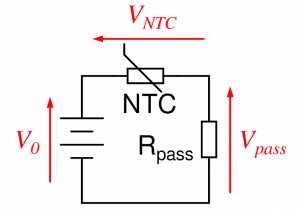Temperature measurements with an NTC thermistorFollowing a discussion on Facebook I put here an online tool to calculate the output of a voltage divider consisting of a fixed resistor and an NTC thermistor following a first order Steinhart–Hart equation.

The calculations are based on a voltage divider where either the voltage over the NTC or over the pass resistor is measured. You can change the values in the script according to your own settings. If you break the script for some reason, just reload the page.

SageMath uses Python as programming language.